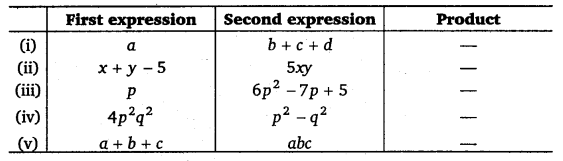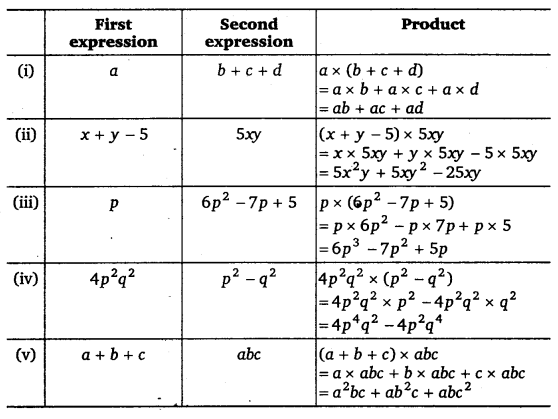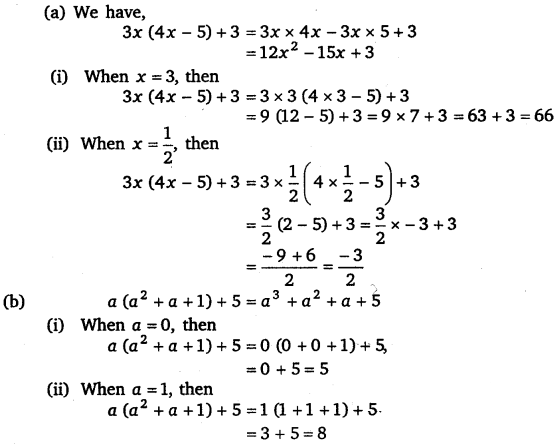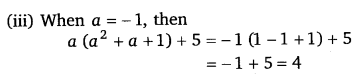# Class 8 Maths NCERT Solutions for Chapter – 9 Algebraic Expressions and Identities Ex – 9.3

### Algebraic Expressions and Identities

Question 1.
Carry out the multiplication of the expressions in each of the following pairs :
(i)
4p, q r + r
(ii) ab, a – -b
(iii) a + b, 7a2b2
(iv) a2– 9, 4a
(v) pq + qr + rp, 0

Solution:
(i) 4p x (q + r) = 4px q + 4p x r
= 4pq + 4pr
(ii) ab x (a – b) = ab x a – ab
= a2b – ab2
(iii) (a + b) x 7a2b2 = a x 7a2b2 + b x 7a2b2
= 7a3b2 + 7a2b3
(iv) (a2 -9)x 4a = a2 x 4a – 9 x 4a
= 4a3 – 36a
(v) (pq + qr + rp) x 0 = 0

Question 2.
Complete the table :Solution:
Completed table is as under :Question 3.
Find the product:Solution:Question 4.
(a) Simplify : 3x (4x – 5) + 3 and find its value for
(i) x = 3,
(ii) x =$\frac { 1 }{ 2 }$
(b) Simplify : a (a2 + a + 1) + 5 and find its value for
(i) a = 0,
(ii) a = 1,
(iii) a = – 1

Solution:Question 5.
(a) Add : p (p – q), q (q – r) and r (r – p)
(b) Add : 2x (z – x – y) and 2y (z – y – x)
(c) Subtract : 31 (1 – 4m + 5n) from 41 (10n – 3m + 21)
(d) Subtract : 3a (a + b + c) – 2b (a – 6 + c) from 4c (-a + b + c)

Solution:#### Class 8 Maths NCERT Solutions for Chapter – 9 Algebraic Expressions and Identities Ex – 9.4

Lorem ipsum dolor sit amet, consectetur adipiscing elit. Phasellus cursus rutrum est nec suscipit. Ut et ultrices nisi. Vivamus id nisl ligula. Nulla sed iaculis ipsum.

Company Name